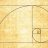# Fib pivot levels

A

#### ArrOne

##### New member
2019 Donor
Is there any indicator out there that plots pivots using fibs?

Fibonacci Pivot Point = (High + Low + Close) / 3
S1 = Fibonacci Pivot – 0.382 * (High – Low)
S2 = Fibonacci Pivot – 0.618 * (High – Low)
S3 = Fibonacci Pivot – 1.000 * (High – Low)
R1 = Fibonacci Pivot + 0.382 * (High – Low)
R2 = Fibonacci Pivot + 0.618 * (High – Low)
R3 = Fibonacci Pivot + 1.000 * (High – Low)#### BenTen

Staff
VIP
Have you tried searching for it on here? I think we posted a few Fibs and Pivots related indicators.#### korygill

##### Active member
VIPCombine a Pivot study with Fib study for double confirmation Questions 0Looking for a different type of Fib Extensions study Questions 0